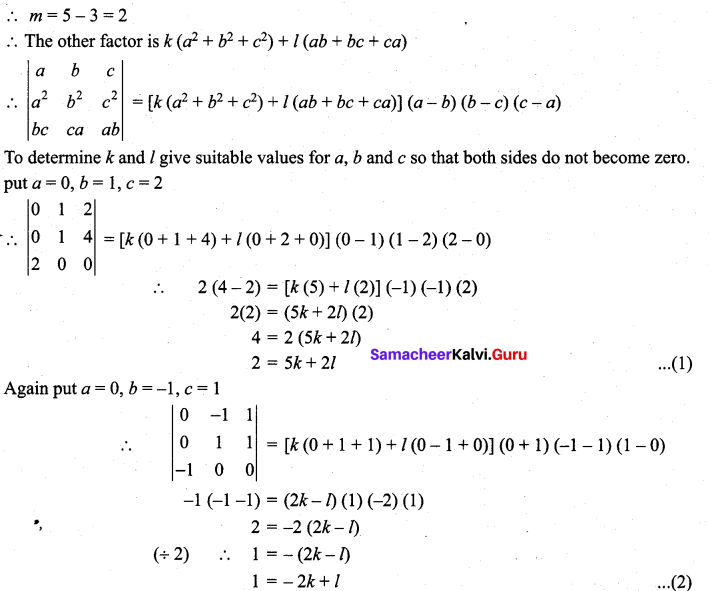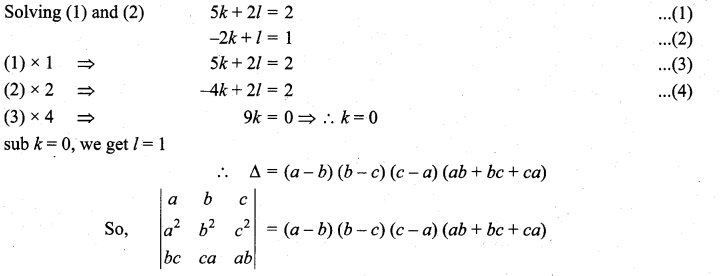# Samacheer Kalvi 11th Maths Solutions Chapter 7 Matrices and Determinants Ex 7.3

## Tamilnadu Samacheer Kalvi 11th Maths Solutions Chapter 7 Matrices and Determinants Ex 7.3

Solve the following problems by using factor theorem

Question 1.
Show that <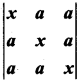= (x – a)2 (x + 2a)
Solution: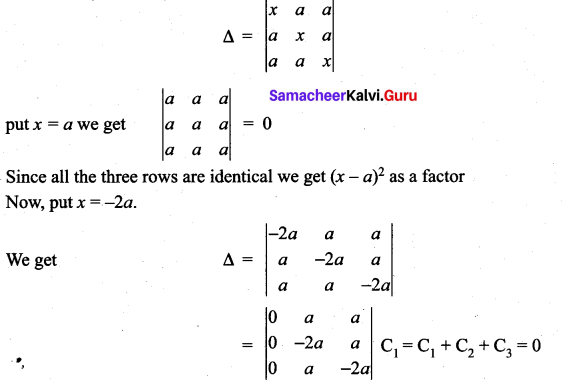⇒ (x + 2d) is a factor of A.
Now degree of Δ is 3 (x × x × x = x3) and we have 3 factors for A
∴ There can be a constant as a factor for A.
(i.e.,) Δ = k(x – a)2 (x + 2d)
equating coefficient of x3 on either sides we get k = 1∴ Δ = (x – a)2 (x + 2a)

Question 2.
Show that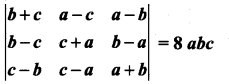Solution: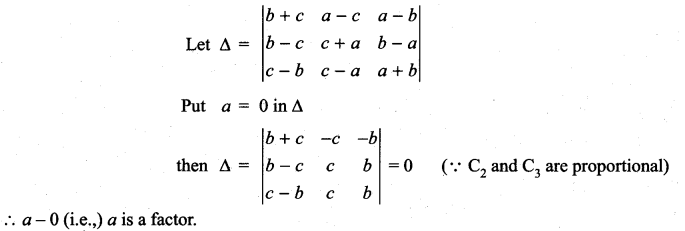Similarly b and c are factors of Δ.
The product of the leading diagonal elements is (b + c) (c + a) (a + b)
The degree is 3. And we got 3 factors for Δ ∴ m = 3 – 3 = 0
∴ there can be a constant k as a factor for Δ.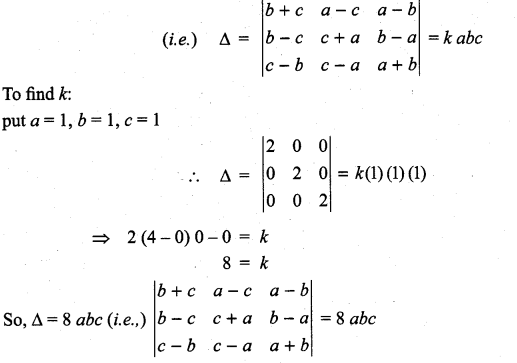>

Question 3.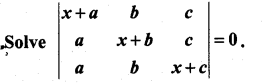Solution: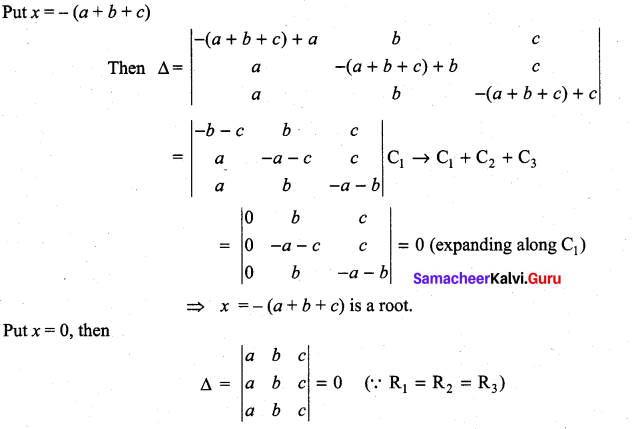⇒ x = 0, 0 are roots.
Now the degree of the leading diagonal elements is 3.
∴ the equation is of degree 3, so the roots are 0, 0, – (a + b + c)Question 4.
Show that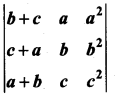= (a + b + c) (a – b) (b – c) (c – a)
Solution: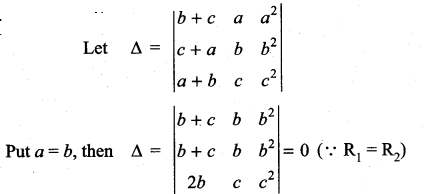⇒ (a – b) is a factor of Δ.
Similarly (b – c) and (c – a) are factors of Δ.
The degree of the product of elements along leading diagonal is 1 + 1 + 2 = 4 and we got 3 factors for Δ. m = 4 – 3 = 1
∴ There can be one more factor symmetric with a, b, c which is of the form k (a + b + c).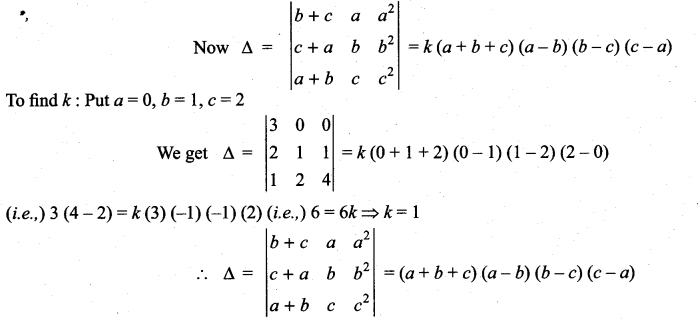Question 5.
Solve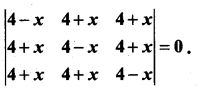Solution: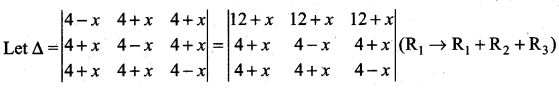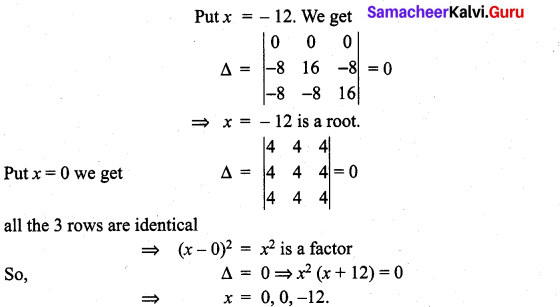Question 6.
Show that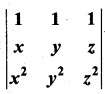= (x – y) (y – z) (z – x)
Solution: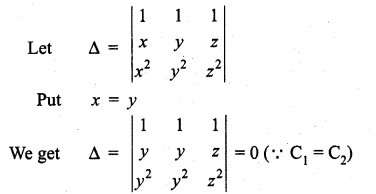⇒ (x – y) is a factor of Δ.
Similarly (y – z) and (z – x) are factors of Δ.
Now degree of Δ = 0 + 1 + 2 = 3 and we have 3 factors of Δ.
and so there can be a constant k as a factor of Δ.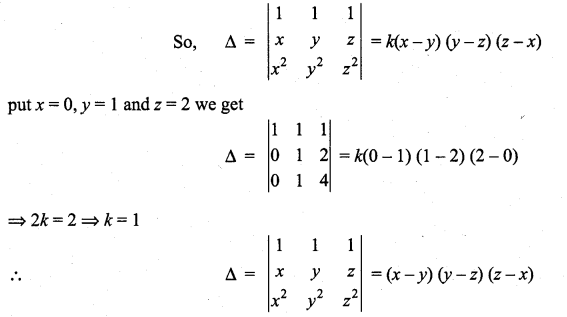### Samacheer Kalvi 11th Maths Solutions Chapter 7 Matrices and Determinants Ex 7.3 Additional Problems

Question 1.
Prove that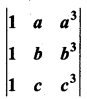= (a – b) (b – c) (c – a) (a + b + c).
Solution: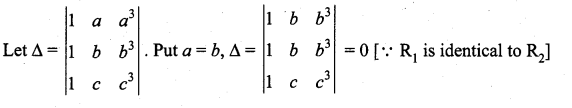∴ (a – b) is a factor of Δ.
Similarly we observe that Δ is symmetric in a, b, c by putting b = c, c = a, we get Δ = 0. Hence (b – c) and (c – a) are also factors of Δ.
∴ The product (a – b) (b – c) (c – a) is a factor of Δ. The degree of this product is 3. The product of leading diagonal elements is 1. bc3. The degree of this product is 4.
∴ By cyclic and symmetric properties, the remaining symmetric factor of first degree must be k (a + b + c), where k is any non-zero constant.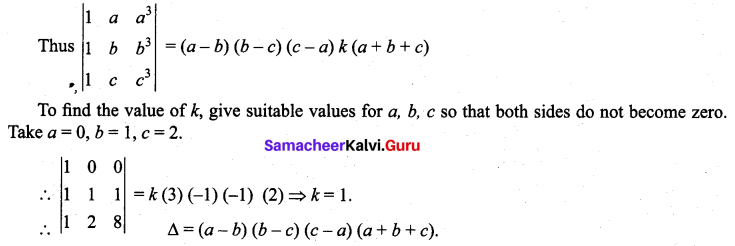Question 2.
Using factor method show that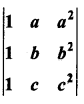= (a – b) (b – c) (c – a)
Solution: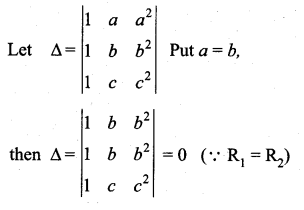⇒ (a – b) is a factor of Δ.
similarly (b – c) and (c – a) are factors of Δ.
The product of leading diagonal elements is bc2. The degree of the product is 1 + 2 = 3.
∴ there will be three factors for Δ. We got 3 factors for Δ as (a – b), (b – c) and (c – a). Its degree = 3. ∴ m = 3 – 3 = 0
∴ there can be a constant k as a factor of Δ.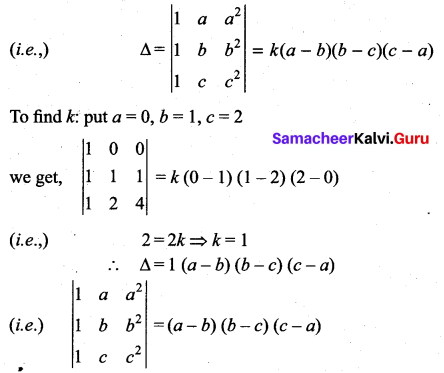Question 3.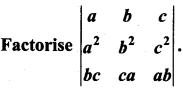Solution: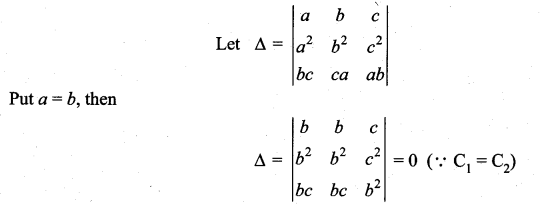⇒ (a – b) is a factor of A.
Similarly (b – c) and (c – a) are factors of Δ.
The degree of Δ = 5 and degree of product of factors = 3.